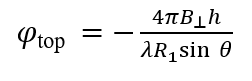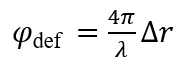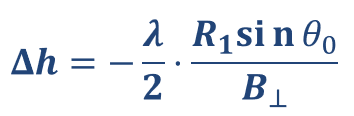# Millimeter Accuracy Using Meter Level DEM

When someone asks you how you get millimeter accuracy results using a 10 or 30 meter DEM during interferogram generation, what is your demystifying answer?

Nice questionInSAR is much more sensitive to deformation than to terrain.

You can look at the expressions for deformation phase and topographic phase：Assuming that the absolute phase difference of two pixels is 2π, which corresponds to exactly one interference fringe, then the corresponding terrain difference and deformation can be deduced according to the above formula:The formula derivation process in different reference materials may be different, but one thing is certain, InSAR deformation measurement is on the order of wavelength (centimeter or even millimeter level), topographic measurements range in magnitude from meters to hundreds of meters.

2 Likes

Nice. But how would you answer this question if asked by someone who doesn’t work with InSAR and maybe only understands basic science?

"SAR Interferometry (InSAR) involves the use of two satellite SAR images acquired over the same area at different times and/or from slightly different acquisition geometries to make an interferogram (…) a differential interferogram is a SAR interferogram compensated for possible phase changes due to different acquisition geometries. In general, differential InSAR analysis aims at measuring surface deformation phenomena by tracking changes in the sensor-to-target distance after removing possible effects due to any other factors "(page 23 from this document).

Therefore DInSAR is a class of techniques that is used to acquire some usefull information from the complex SAR image. If you want to extract displacement, you should adopt one of multiple multi-interferogram tecnhiques as mentioned in page 29 of previous document (PSI, SBAS, etc).

Understanding how the DEM accuracy affects results will depend on the multi_interferogram technique you will use.

Nevertheless the accuracy is not that much relevant (if you a have a prior DEM, it will be used mainly for co-regstration purposes, “in the minimum one could consider the Earth ellipsoid as an a priori model” ) becuase if you take a look to equation 4.5 of this document as to eq.4 from page 37 of EGMS document, you will find that phase error due to topographic accuracy (or the DEM error) will be estimated during the process of LOS displacement estimation (in mm, although the DEM error will be in meters).

As it is mentioned on page 57 of the ESA document, therefore « we can estimate both the residual elevation and the mean line of sight velocity, provided that the signal-to-noise ratio is high enough».

Reason why you should have multiple interferograms to improve your accury results, either for DEM residues or to LOS displacement.

1 Like